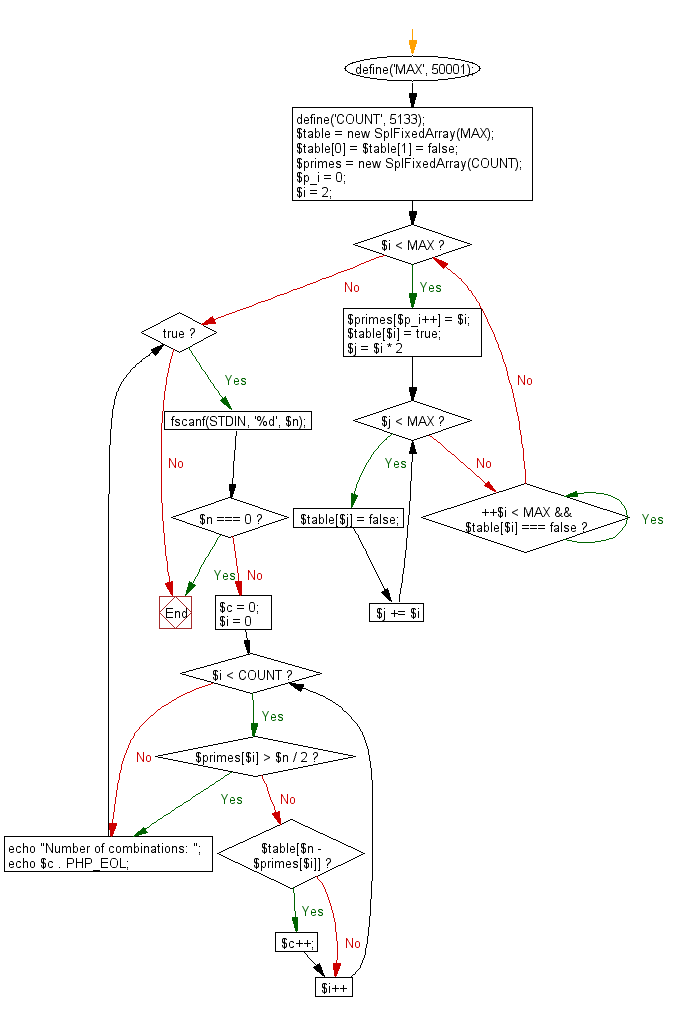﻿ PHP Exercise: Print the number of combinations - w3resource

# PHP Exercises: Print the number of combinations

## PHP: Exercise-66 with Solution

Write a PHP program that accept an even number (n should be greater than or equal to 4 and less than or equal to 50000, Goldbach number) from the user and create a combinations that express the given number as a sum of two prime numbers. Print the number of combinations.
Goldbach number: A Goldbach number is a positive even integer that can be expressed as the sum of two odd primes. Since four is the only even number greater than two that requires the even prime 2 in order to be written as the sum of two primes, another form of the statement of Goldbach's conjecture is that all even integers greater than 4 are Goldbach numbers.
The expression of a given even number as a sum of two primes is called a Goldbach partition of that number. The following are examples of Goldbach partitions for some even numbers:
6 = 3 + 3
8 = 3 + 5
10 = 3 + 7 = 5 + 5
12 = 7 + 5
...
100 = 3 + 97 = 11 + 89 = 17 + 83 = 29 + 71 = 41 + 59 = 47 + 53.

Input: n ( n ≤ 10000). Input 0 to exit the program.

Sample Solution: -

PHP Code:

``````<?php
define('MAX', 50001);
define('COUNT', 5133);
\$table = new SplFixedArray(MAX);
\$table = \$table = false;
\$primes = new SplFixedArray(COUNT);
\$p_i = 0;
\$i = 2;
while (\$i < MAX) {
\$primes[\$p_i++] = \$i;
\$table[\$i] = true;
for (\$j = \$i * 2; \$j < MAX; \$j += \$i) {
\$table[\$j] = false;
}
while (++\$i < MAX && \$table[\$i] === false);
}
while (true) {
fscanf(STDIN, '%d', \$n);
if (\$n === 0) {
break;
}
\$c = 0;
for (\$i = 0; \$i < COUNT; \$i++) {
if (\$primes[\$i] > \$n / 2) {
break;
}
if (\$table[\$n - \$primes[\$i]]) {
\$c++;
}
}
echo "Number of combinations: ";
echo \$c . PHP_EOL;
}

?>
```
```

Sample Input:
100
0

Sample Output:

`Number of combinations: 6`

Flowchart:PHP Code Editor:

Have another way to solve this solution? Contribute your code (and comments) through Disqus.

What is the difficulty level of this exercise?

Test your Programming skills with w3resource's quiz.

﻿

## PHP: Tips of the Day

Returns all elements in an array except for the first one

Example:

```<?php
function tips_tail(\$items)
{
return count(\$items) > 1 ? array_slice(\$items, 1) : \$items;
}

print_r(tips_tail([1, 5, 7]));
?>
```

Output:

```Array
(
 => 5
 => 7
)
```